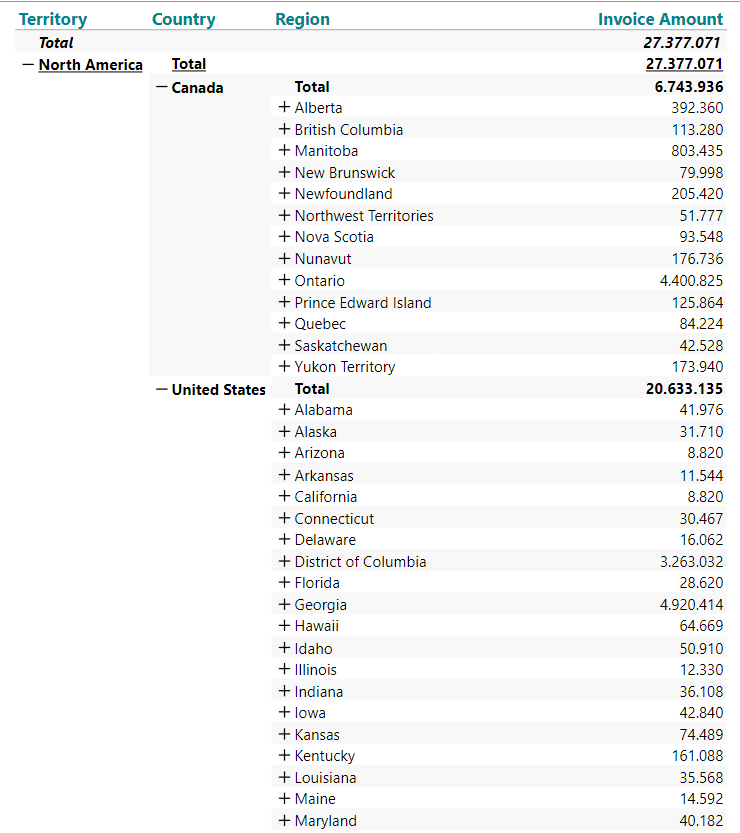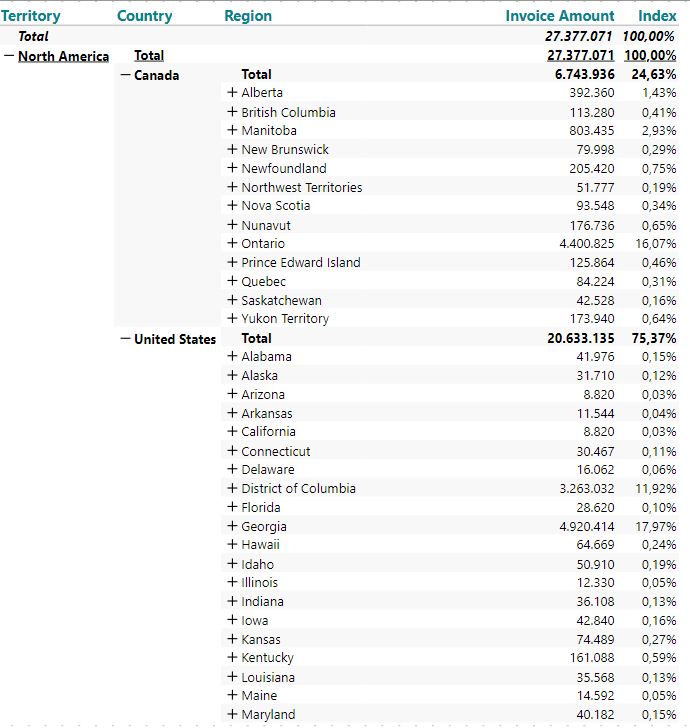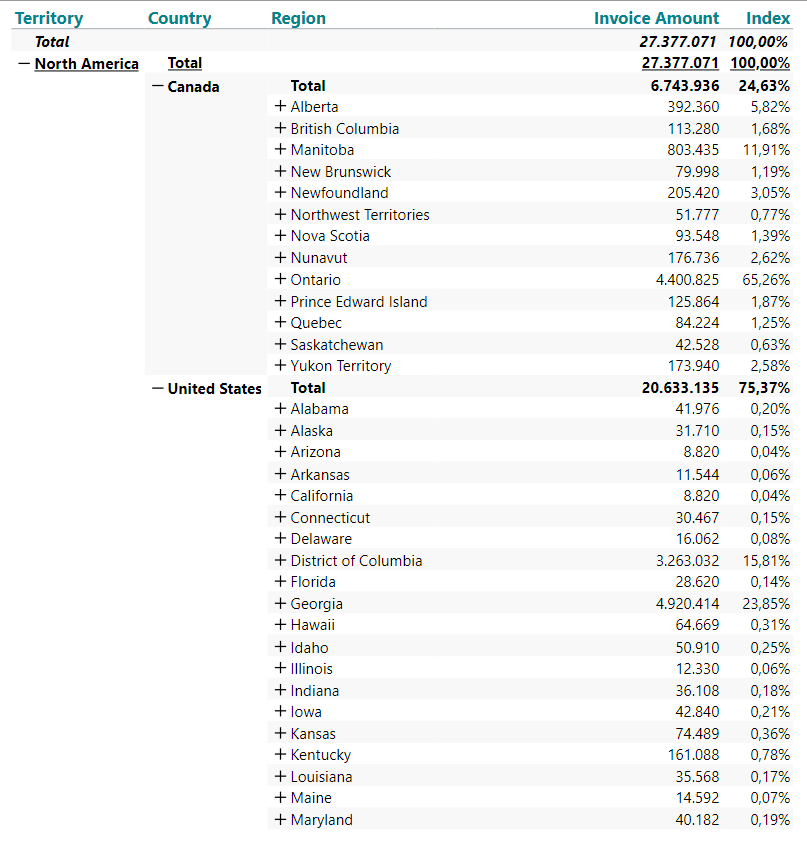# December 14th: Creating a "local" index in a hierarchy

The calculations in TARGIT offers a number of modifiers that can help you when working with hierarchies.

One of those is the sibling (s) modifier which comes in really handy when you want to calculation something "locally" in a hierarchy.

Here's an example of a hierarchy showing Invoice Amount by Territory-Country-Region:If you wanted to know how much a certain region contributed to the total, you might add a calculated column called index with a syntax like this:

sum(d1,0,m1)/sum(d1,all,m1)

If then format that number as a percent, it could look like this:Every percentage shows how much a certain region contributes compared to the sum of all regions in all countries in the territory.

But what if you wanted to make this local?

So now you want the e.g. Alabama index to be calculated against the United States numbers and not the grand total.

A very, very simple addition to the syntax will do the trick:

sum(d1,0,m1)/sum(d1,all(s),m1)

Adding the s as a modifier to all, means all siblings (all regions within a certain country).

The result is a local index: